Practice Test: Civil Engineering (CE)- 12

# Practice Test: Civil Engineering (CE)- 12

Test Description

## 65 Questions MCQ Test GATE Civil Engineering (CE) 2023 Mock Test Series | Practice Test: Civil Engineering (CE)- 12

Practice Test: Civil Engineering (CE)- 12 for Civil Engineering (CE) 2023 is part of GATE Civil Engineering (CE) 2023 Mock Test Series preparation. The Practice Test: Civil Engineering (CE)- 12 questions and answers have been prepared according to the Civil Engineering (CE) exam syllabus.The Practice Test: Civil Engineering (CE)- 12 MCQs are made for Civil Engineering (CE) 2023 Exam. Find important definitions, questions, notes, meanings, examples, exercises, MCQs and online tests for Practice Test: Civil Engineering (CE)- 12 below.
Solutions of Practice Test: Civil Engineering (CE)- 12 questions in English are available as part of our GATE Civil Engineering (CE) 2023 Mock Test Series for Civil Engineering (CE) & Practice Test: Civil Engineering (CE)- 12 solutions in Hindi for GATE Civil Engineering (CE) 2023 Mock Test Series course. Download more important topics, notes, lectures and mock test series for Civil Engineering (CE) Exam by signing up for free. Attempt Practice Test: Civil Engineering (CE)- 12 | 65 questions in 180 minutes | Mock test for Civil Engineering (CE) preparation | Free important questions MCQ to study GATE Civil Engineering (CE) 2023 Mock Test Series for Civil Engineering (CE) Exam | Download free PDF with solutions
 1 Crore+ students have signed up on EduRev. Have you?
Practice Test: Civil Engineering (CE)- 12 - Question 1

### In the following question, four words are given out of which one word is incorrectly spelt. Select the incorrectly spelt word

Detailed Solution for Practice Test: Civil Engineering (CE)- 12 - Question 1 Option B has the misspelt word. The correct spelling of the word is "Insatiable" which means unquenchable. The meanings of other words are

Flounder=> suffer mentally

Anoint=> Nominate or choose (someone) as successor to or leading candidate for a position

Maneuver=> Move, ploy, manipulate

Practice Test: Civil Engineering (CE)- 12 - Question 2

### The smallest angle of a triangle is equal to two thirds of the smallest angle of a quadrilateral. The ratio between the angles of the quadrilateral is 3: 4: 5: 6. The largest angle of the triangle is twice its smallest angle. What is the sum, in degrees, of the second largest angle of the triangle and the largest angle of the quadrilateral?

Detailed Solution for Practice Test: Civil Engineering (CE)- 12 - Question 2 Let the angles of quadrilateral are 3x, 4x, 5x, 6x

So, 3x + 4x + 5x + 6x = 360

x = 20

Smallest angle of quadrilateral = 3 × 20 = 60 °

Smallest angle of triangle = 2/3 ×60= 40∘

Largest angle of triangle = 2 × 40 ° = 60 °

Three angles of triangle are 40 °, 60 °, 80 °

Largest angle of quadrilateral is 120 °

Sum (2nd largest angle of triangle + largest angle of quadrilateral)

= 60 ° + 120 ° = 180 °.

Practice Test: Civil Engineering (CE)- 12 - Question 3

### If x : y = 3 : 2, then the ratio 2x2 + 3y2 : 3x2 - 2y2 is equal to

Detailed Solution for Practice Test: Civil Engineering (CE)- 12 - Question 3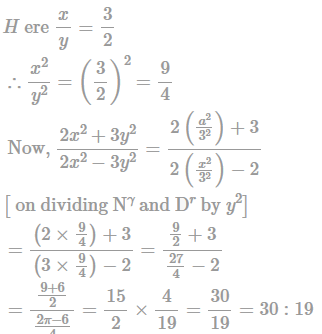Practice Test: Civil Engineering (CE)- 12 - Question 4

In the following question, out of the five alternatives, select the word similar in meaning to the given word/phrase.

Detailed Solution for Practice Test: Civil Engineering (CE)- 12 - Question 4 Somniloquism = the tendency to talk in one's sleep.

Somnambulism = the habit of walking in one's sleep.

Obsession = something or someone that you think about all the time.

Hallucination = a sensory experience of something that does not exist outside the mind.

Soliloquy = an act of speaking one's thoughts aloud when by oneself or regardless of any hearers, especially by a character in a play.

So, the correct word is "obsession".

Practice Test: Civil Engineering (CE)- 12 - Question 5

In the following question, out of the four alternatives, select the word opposite in meaning to the given word.

Detailed Solution for Practice Test: Civil Engineering (CE)- 12 - Question 5 Adipose refers to fatty. Contrary to that, thin is the most suitable response.

corpulent = fat, obese

glutinous = viscose, sticky

oleaginous = resembling or having the properties of oil

Practice Test: Civil Engineering (CE)- 12 - Question 6

Select the related word/letters/number from the given alternatives.

Psychology : Mind :: Arithmetic : ?

Detailed Solution for Practice Test: Civil Engineering (CE)- 12 - Question 6

This problem is based on the subject and its area of study.

Psychology is the science or study of the mind. Arithmetic is the branch of Mathematics that deals with computing of numbers (Addition, Subtraction, Multiplication and Division).

Hence, option B is the right answer.

Practice Test: Civil Engineering (CE)- 12 - Question 7

Select the most appropriate option to fill in the blank.

As he could not execute the work properly, he had no option _____ to leave the organization.

Detailed Solution for Practice Test: Civil Engineering (CE)- 12 - Question 7

"No option" is generally followed by the conjunction "but". Therefore, option D is the correct answer. See some examples

I had no option but to open the gate.

She has no option but to quit her studies.

Practice Test: Civil Engineering (CE)- 12 - Question 8

In a triangle ABC, angle A = 60o , angle B = 100o and AB = 10cm. find AC.

Detailed Solution for Practice Test: Civil Engineering (CE)- 12 - Question 8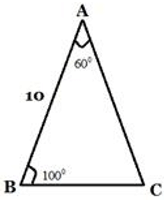By angle sum property angle C= 20 degree

as we know from sin formula

SinA/BC= SinB/AC= SinC/AB

Sin 100°/ AC= Sin 20°/ 10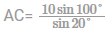Practice Test: Civil Engineering (CE)- 12 - Question 9

What is the equation of the line which passes through the points (-1,2) and (4,-3)?

Detailed Solution for Practice Test: Civil Engineering (CE)- 12 - Question 9

Equation of a line passing through two given paints is given by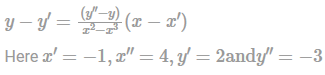Therefore, equation of the line passing through these two paints is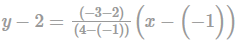⇒ y−2 = −1(x+1)

⇒ x + y = 1

Practice Test: Civil Engineering (CE)- 12 - Question 10

P and Q can do a project in 25 and 50 days respectively. In how many days can they complete 18% of the project if they work together?

Detailed Solution for Practice Test: Civil Engineering (CE)- 12 - Question 10

According to the question

P and Q can do a project in 25 and 50 days respectively.

Let us assume total unit of work available is 50 (LCM of 25 & 50)

And, P does 2 unit of work daily to get it completed in 25 days

Likewise, Q does 1 unit of work daily.

Therefore, total work done by them in 1 day=3 unit

Now, no. of days requires to complete 18% of the project if they work together

⇒ 18% of 50) / 3

⇒ 9/3=3 days

Hence the correct answer is option B

Practice Test: Civil Engineering (CE)- 12 - Question 11

If the median of (x-1), (x-1), (x-4), (x+4), (x-3) is zero then the value of x is ________?

Detailed Solution for Practice Test: Civil Engineering (CE)- 12 - Question 11 Arrange in ascending order

I.e. x-4, x-3, x-1, x+1, x+4

Now x-1 = 0

X = 1

*Answer can only contain numeric values
Practice Test: Civil Engineering (CE)- 12 - Question 12

A box contains 2 identical bags A and B . Bag A contains 2 Red and 5 Green balls. Bag B contains 2 Red and 6 Green Balls. A person draws a ball at random. If the drawn ball was Red what is the probability that it was from bag A?

Detailed Solution for Practice Test: Civil Engineering (CE)- 12 - Question 12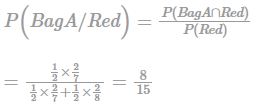Practice Test: Civil Engineering (CE)- 12 - Question 13

A square matrix B is skew symmetric if

Detailed Solution for Practice Test: Civil Engineering (CE)- 12 - Question 13 Skew-symmetric (or antisymmetric or antimetric)matrix is a square matrix whose transpose is its negation; that is, it satisfies the condition −A = AT.
Practice Test: Civil Engineering (CE)- 12 - Question 14

If 2x - 3y = 24 and 3x + 4y = 2, find the value of x - y?

Detailed Solution for Practice Test: Civil Engineering (CE)- 12 - Question 14 In a pair of linear equations

2x−3y = 24−−x−x−z−(1)

3x+4y = 2−x−z−z−(2)

If we multiply (1) by 4 and (2) by 3, we get 8x−12y = 96−−x−y−(3)

9x+12y = 6−x−−(4)

Now adding equation (3) and (4), we get 17x = 102

x = 102÷17

x = 6

On substituting x=6 in (1), we get y as:

12−3y = 24

−3y = 12

y = −4

Since values of x and y are known, so finding value of x−y will result in:

x−y = 6−(−4) = 6+4 = 10

x−y = 10, so correct option is ©

Practice Test: Civil Engineering (CE)- 12 - Question 15

If A and B are invertible matrices, then inverse of AB is:

Detailed Solution for Practice Test: Civil Engineering (CE)- 12 - Question 15 (AB)(B-1A-1) = A(BB-1)A-1

= (A1)A-1 = AA-1 = 1

Also, (B-1 A‑1) (AB) = 1

(AB)-1 = B-1 A-1

*Answer can only contain numeric values
Practice Test: Civil Engineering (CE)- 12 - Question 16

A 400g/L solution of common salt was discharged into a stream at a constant rate of 45l/s. At a d/s section where salt solution is known to have completely mixed with the stream flow equilibrium concentration was read as 100 ppm. If a background concentration of 18ppm is applicable, then, the discharge in the stream in m3/s is ___________.

Detailed Solution for Practice Test: Civil Engineering (CE)- 12 - Question 16 By constant rate injection method,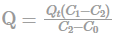Qt = 45I/s=0.045m3/s

C1 = 0.4

C2 = 0.0001

C0 = 0.000018

∴ Q = 0.045(0.4−0.0001)/0.0001−0.000018 = 219.45m3/s

Practice Test: Civil Engineering (CE)- 12 - Question 17

Find the maximum bending moment occuring at the point C due to a moving uniform load of 2 kN/m having a length of 3 m.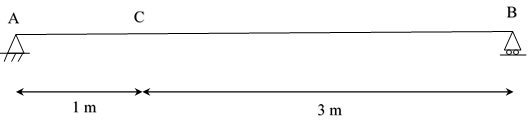Detailed Solution for Practice Test: Civil Engineering (CE)- 12 - Question 17 Drawing the bending moment ILD,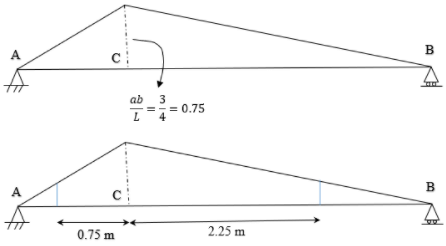Maximum bending moment occurs at C when it divides the load in the same ratio as it divides the span, i.e. 1: 3 So total bending moment, area of ILD multiplied with load

[1/2 × (0.1875 + 0.75) × 0.75 + 1/2 × (0.1875 + 0.75) × 2.25] × 2

= [12 × (0.1875+0.75)×3] × 2 = 2.8125kNm

Practice Test: Civil Engineering (CE)- 12 - Question 18

A prestressed concrete beam of size 300 mm × 900 mm is prestressed with an initial prestressing force of 810 kN at an eccentricity of 360 mm at mid-span. Stress at the top fibre due prestress alone

Detailed Solution for Practice Test: Civil Engineering (CE)- 12 - Question 18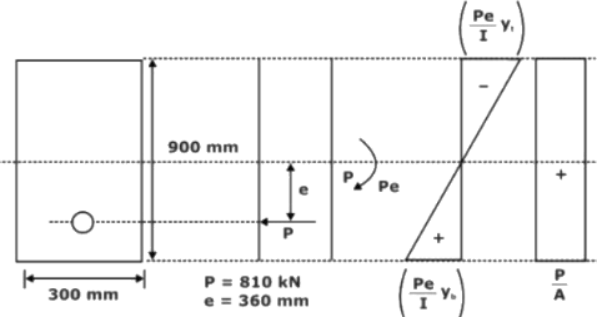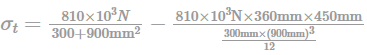= 3MPa − 7.2MPa

= −4.2N/mm2

Practice Test: Civil Engineering (CE)- 12 - Question 19

For two cycles coupled in series, the topping cycle has an efficiency of 40% and the bottoming cycle has an efficiency of 30%. The overall combined cycle efficiency is:

Detailed Solution for Practice Test: Civil Engineering (CE)- 12 - Question 19 η = η1 + η2 − η1 × η2

η = 0.40 + 0.30 − 0.4 × 0.3

η = 58%

Hence, the correct answer is 58%.

Practice Test: Civil Engineering (CE)- 12 - Question 20

The slope at point B is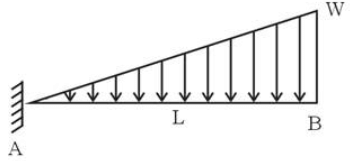Detailed Solution for Practice Test: Civil Engineering (CE)- 12 - Question 20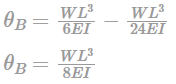Practice Test: Civil Engineering (CE)- 12 - Question 21

Which of the following statements is incorrect?

Detailed Solution for Practice Test: Civil Engineering (CE)- 12 - Question 21 Light weight concrete has density less than 1930 kg/m3. Other statements are correct.
Practice Test: Civil Engineering (CE)- 12 - Question 22

A horizontal jet of water with its cross–sectional area of 0.0028 m2 hits a fixed vertical plate with a velocity of 5 m/s. After impact the jet splits symmetrically in a plane parallel to the plane of the plate. The force of impact (in N) of the jet on the plate is

Detailed Solution for Practice Test: Civil Engineering (CE)- 12 - Question 22 The Jet impacts plate as shown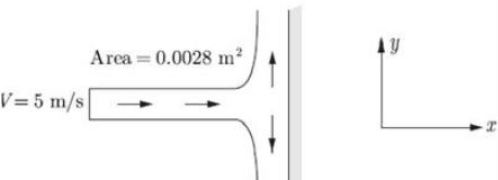the velocity in x direction becomes zero after impact so using impulse momentum theorem

F = ρ0Q1V1 − ρωQ2V2 = ρωQ(V1−0)

Q = AV = 0.0028 × 5 = 0.014 ρω = 1000kg/m3

So ForceF = 1000 × 0.014 × (5−0)

= 70N

Practice Test: Civil Engineering (CE)- 12 - Question 23

i. The minimum spacing required for the longitudinal reinforcement increases as the grade of steel increases.

ii. Less strain is required due to the high grade of steel.

Which of the above statements are incorrect?

Detailed Solution for Practice Test: Civil Engineering (CE)- 12 - Question 23 More strain is required to yield high grade steel. So only ii is incorrect.
Practice Test: Civil Engineering (CE)- 12 - Question 24

A portal frame is loaded as shown. Find the angle θB if it has a sway of 12.5/EI.

(Consider usual sign convention)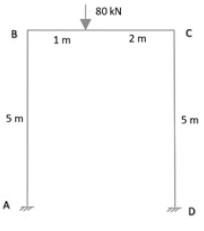Detailed Solution for Practice Test: Civil Engineering (CE)- 12 - Question 24 Fixed end moments are zero.

MFAB = MFBA = 0

By slope deflection equation, MAB = MFAB + 2EIL(2θAB−3Δ/L)

But the sway is towards left, so Δ is negative, 0 = 2EI/5(θB + 3×12.5/5×EI)

θB = −3×12.5/5×EI

θB = −7.5/EI

Practice Test: Civil Engineering (CE)- 12 - Question 25

Which of the following statements are true?

1) Slope deflection method is always used for determinate structures.

2) Slope deflection method is the displacement method.

3) Modified slope deflection equation is used when one support is hinged.

4) Superposition cannot be applied in slope deflection equations.

Detailed Solution for Practice Test: Civil Engineering (CE)- 12 - Question 25 The statements 2 and 3 are true. Statement 4 is false because the slope deflection method is derived from the superposition method.
Practice Test: Civil Engineering (CE)- 12 - Question 26

Efflorescence of the cement is due to the excess of

Detailed Solution for Practice Test: Civil Engineering (CE)- 12 - Question 26 Efflorescence (white patches on the surface) are due to the excess of alkalis in cement.
Practice Test: Civil Engineering (CE)- 12 - Question 27

Match the following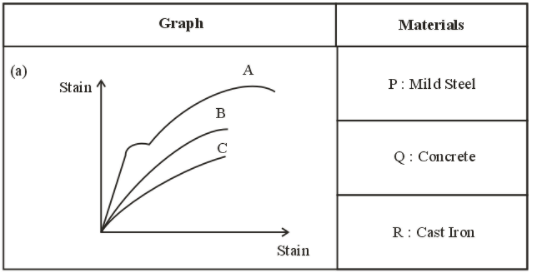Detailed Solution for Practice Test: Civil Engineering (CE)- 12 - Question 27 Mild steel is ductile. So, it has an elastic limit point(limit of proportionality).

Cast iron and Concrete are Brittle, but Concrete has less strength than Cast Iron.

Practice Test: Civil Engineering (CE)- 12 - Question 28

A bar of circular cross-section is clamped at ends P and Q as shown in the figure. A torsional moment T = 150 Nm is applied at a distance of 100mm from end P. The torsional reactions (Tp, T0) in Nm at the ends P and Q respectively are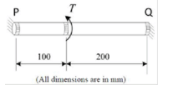Detailed Solution for Practice Test: Civil Engineering (CE)- 12 - Question 28 Since, G and are constant for bar,

T1L1 = T2L2

T1.100 = T2.200

T1 = 2T2

And, T1 + T2 = T

Hence, T1 = 100 Nm and T2 = 50Nm

Practice Test: Civil Engineering (CE)- 12 - Question 29

A stepped cantilever is subjected to moments as shown. The vertical deflection at free end is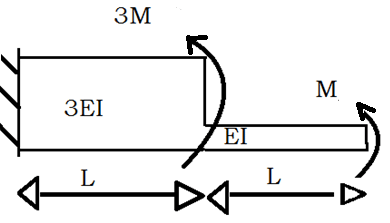Detailed Solution for Practice Test: Civil Engineering (CE)- 12 - Question 29 Drawing M/EI diagram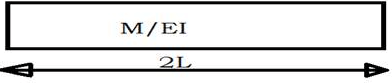Deflection of free end with respect to fixed end= Moment of M/EI diagram between the ends.

δ = M/EI × 2L × L.

δ = 2ML2/EI

*Answer can only contain numeric values
Practice Test: Civil Engineering (CE)- 12 - Question 30

A square plate of dimension L × L is subjected to a uniform pressure load p = 250 MPa on its edges as shown in the figure. Assume plane stress conditions. The Young’s modulus E = 200 GPa.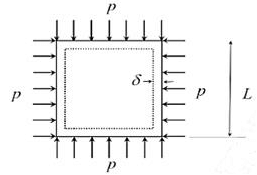The deformed shape is a square of dimension L = 2δ. If L =2m and δ =0.001 m, the Poisson’s ratio of the plate material is ____.

Detailed Solution for Practice Test: Civil Engineering (CE)- 12 - Question 30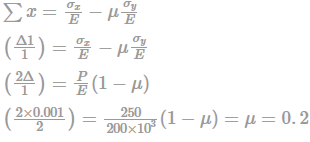Practice Test: Civil Engineering (CE)- 12 - Question 31

A slow-moving vehicle at a speed of 84 kmph requires a clear spacing of _____ (in m) to overtake.

Detailed Solution for Practice Test: Civil Engineering (CE)- 12 - Question 31 The clear spacing or the gap required by the overtaken vehicle is computed using the following relationship.

S = 0.7 Vb + 6 m

Vb = (84 x 1000)/(60 x 60) = 23.33 m/s

Clear space/ space headway = 16.33 + 6 = 22.33 m

Practice Test: Civil Engineering (CE)- 12 - Question 32

The runway length after correcting for elevation and temperature is 2800m. if the effective gradient on runway is 0.8% then revised runway length will be?

Detailed Solution for Practice Test: Civil Engineering (CE)- 12 - Question 32 Correction for gradient

= 20/100 × 2800 × 0.8 = 448

Corrected length = 2800 + 448 = 3248 m

Practice Test: Civil Engineering (CE)- 12 - Question 33

A large hydraulic turbine is to generate 300 kW at 1000 rpm under a head of 40 m. for initial testing, a 1:4 scale model of the turbine operates under a head of 10 m. the power generated by the model (in kW) will be

Detailed Solution for Practice Test: Civil Engineering (CE)- 12 - Question 33 For similar turbines specific power will be same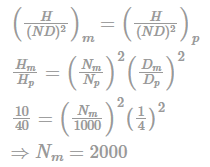Now specific speed will be same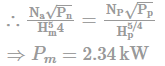Practice Test: Civil Engineering (CE)- 12 - Question 34

Consider the following statements:

1) The alkalinity of the fresh water is alkaline but as time passes it becomes acidic.

2) The fresh domestic waste water is dark in colour but as time passes it becomes grey.

Detailed Solution for Practice Test: Civil Engineering (CE)- 12 - Question 34 The fresh water is alkaline but becomes acidic after some time due to bacterial action. The fresh water is grey in colour which becomes dark or black in colour due to decomposition and reduction of oxygen to zero in stale water. Only statement I is correct.
Practice Test: Civil Engineering (CE)- 12 - Question 35

The colour of the waste water gets converted to dark because of

Detailed Solution for Practice Test: Civil Engineering (CE)- 12 - Question 35 The colour of the fresh waste water gets converted into dark due to the breaking down of organic compounds by bacteria and thus removal of dissolved oxygen in the water.
Practice Test: Civil Engineering (CE)- 12 - Question 36

An ice cream factory has a daily discharge of the waste water at the average rate of 2400 m3/day. The waste water coming out of the factory was tested at 2% dilution and following results were obtained:

DO of aerated water = 5mg/l

DO of diluted sample = 0.5 mg/l

DO of diluted sample after 5 days = 1.4 mg/l

Deoxygenation constant = 0.1 day-1

The ultimate BOD of the sample is

Detailed Solution for Practice Test: Civil Engineering (CE)- 12 - Question 36

4.91-1.4/2 × 100 = 175.5 mg/Id sample,

5×98+0.5×2/100 = 4.91 mg/1

DO of sample after 5 days = 3.4 mg/l

BOD5 of the sample,

4.91-1.4/2 × 100 = 175.5 mg/I

BOD5 = BODu(1-10-kdt)

BODu = 175.5/(1-10-0.5) = 256.66 mg/I

*Answer can only contain numeric values
Practice Test: Civil Engineering (CE)- 12 - Question 37

Treatment plant treating 15MLD of water requires 35mg/l of Alum. If the water has 10mg/l of alkalinity as CaCO3, find the quantity of quick lime required (tons per year as CaO)?

[Al=27, S=32, O=16, H=1, Ca=40, C=12)?

Detailed Solution for Practice Test: Civil Engineering (CE)- 12 - Question 37 666g of Alum = 366g of HCO3-

1mg of alum = 0.45mg of alkalinity as CaCO3

35mg/l of alum = 0.45 × 35 = 15.75 mg/l as CaCO3

Alkalinity to be added additionally = 15.75 – 10 = 5.75 mg/l as CaCO3

Alkaline to be added as CaO = 5.75 × 0.56 =3.22 mg/l

Total quick lime required per year = 3.22 ×15 × 365/1000

= 17.63 ton

*Answer can only contain numeric values
Practice Test: Civil Engineering (CE)- 12 - Question 38

What will be the efficiency (%) of a 20m diameter and 1m deep single state high rate trickling filter having sewage low as 4.5MLD, recirculation rate = 1.4 and BOD influent is about 185mg/l?

Detailed Solution for Practice Test: Civil Engineering (CE)- 12 - Question 38 We have,

Efficiency,

n = 100 / (1+0.0044 (Q Yi/VF)0.5)

F = (1+R/I)/(1+0.1R/I)2 = (1+1.4)/ (1+0.1×1.4)2

= 1.87

QYi = 4.5 × 185

= 832.5 Kg

V = πD2 × 1/4 = 0.0314 ha-m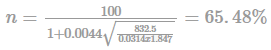Practice Test: Civil Engineering (CE)- 12 - Question 39

The T-alkalinity in water is 400mg/l as CaCO3 and P-alkalinity is 250mg/l as CaCO3. What will be the concentrations of OH-, CO3-2, HCO3- in mg/l respectively?

Detailed Solution for Practice Test: Civil Engineering (CE)- 12 - Question 39

As P > 0.5T; then we have

OH- conc = 2P-T

= 2 × 250-400 = 100mg/l

CO3-2 conc = 2T-2P

= 2×400 -2×250 = 300mg/l

HCO3- conc = 0 mg/l

Note: T alkalinity is the measurement of all species of alkalinity in the water. It is the final endpoint for the alkalinity titration.

P alkalinity or Phenolphthalein alkalinity is the measurement of amount of carbonate and hydroxide using titration water samples with acid of a known concentration and using phenolphthalein indicator. It is measured down to a pH of 8.3.

Practice Test: Civil Engineering (CE)- 12 - Question 40

For a vehicle moving on a single lane road with two-way traffic at a speed of 80 km/h, the Head light distance is _______ m.

Detailed Solution for Practice Test: Civil Engineering (CE)- 12 - Question 40 Headlight distance = Stopping Sight Distance

SSD in case of Single lane with two-way traffic is Twice the SSD

SSD/HSD = 0.278 vt + v2/ (254f)

Take reaction time, t = 2.5 sec

Coefficient of friction, f = 0.35

On substituting, SSD = 55.6 + 71.99 = 127.59 = 128 m

Here HSD = 2 SSD = 256 m

Practice Test: Civil Engineering (CE)- 12 - Question 41

For a fully developed laminar flow of water (dynamic viscosity 0.001 Pa-s) through a pipe of radius 5 cm. the axial pressure gradient is —10 Pa/m. The magnitude or axial velocity (in m/s) at a radial location of 0.2 cm is ___________ m/sec

Detailed Solution for Practice Test: Civil Engineering (CE)- 12 - Question 41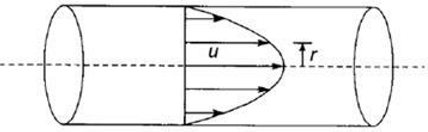For incompressible laminar flows through pipes velocity distribution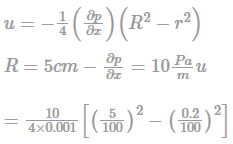= 6.24m/sec.

Practice Test: Civil Engineering (CE)- 12 - Question 42

The rank of the matrix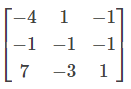is

Detailed Solution for Practice Test: Civil Engineering (CE)- 12 - Question 42

Converting the matrix into Echelon form,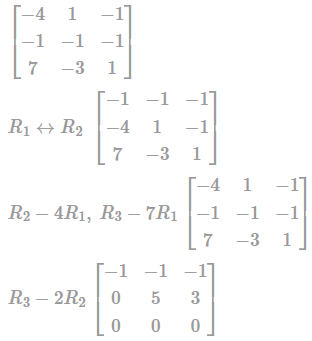Now since the matrix is in Echelon

Rank = Number of no-zero rows = 2

Practice Test: Civil Engineering (CE)- 12 - Question 43

A rod of length L having uniform cross-sectional area A is subjected to a tensile force P as shown in the figure below. If the Young’s modulus of the material varies linearly from E1 to E2 along the length of the rod, the normal stress developed at the section-SS is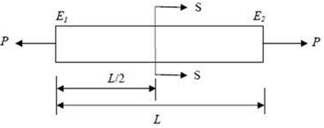Detailed Solution for Practice Test: Civil Engineering (CE)- 12 - Question 43

The normal stress across any section depends only on external force (P) & cross-section area σ = P/A is independent of young's of material.

Practice Test: Civil Engineering (CE)- 12 - Question 44

Given that one root of the equation x3 –10x2 + 31x –30 = 0 is 5, the other two roots are

Detailed Solution for Practice Test: Civil Engineering (CE)- 12 - Question 44

Given, x3-10x2+31x-30=

It's one root is 5, hence

(x-5) (x2-5x+6) = 0

⇒ (x-5) (x2-3x-2x+6) = 0

⇒ (x-5) (x-3)(x-2) = 0

⇒ x = 5,3,2

Practice Test: Civil Engineering (CE)- 12 - Question 45

A steel column section ISHB 350 (width of flange is 250mm and thickness of flange 11.6mm) has to carry a factored load of 2400kN. The yield stress and the ultimate tensile stress of steel and welds are 250MPa and 410MPa respectively and partial safety factor governed by yield and ultimate tensile strength respectively are 1.10 and 1.25. The bearing strength of M25 grade concrete in limit state method of design as per IS 456:2000(inN/mm2) is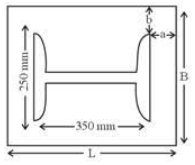Detailed Solution for Practice Test: Civil Engineering (CE)- 12 - Question 45

For ISHB 350 steel column,

bf = 250mm and tf = 11.6mm.

P = 2400kN, fy = 250 MPa.

For M25 grade concrete Fck = 25N/mm2

Bearing strenght of concrete = 0.45fck = 11.25N/mm2

Practice Test: Civil Engineering (CE)- 12 - Question 46

The magnitude of bending moment at fixed end of beam shown in figure above is _________ KNm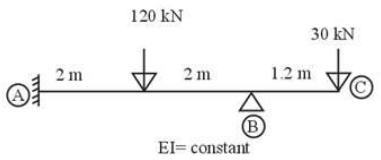Detailed Solution for Practice Test: Civil Engineering (CE)- 12 - Question 46

Calculation of FEM

MFBC = - 30 × 1.2 = -36KNmMFAB

= - 120 × 4 / 8 = -60KNmFBA

= 120 × 4/8

= 60KNm

Distribution factor: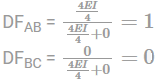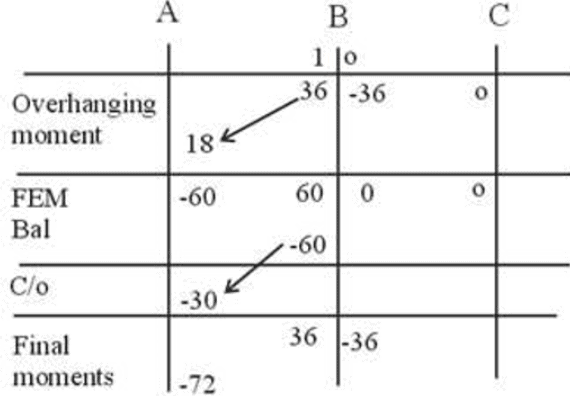FINAL MOMENT AT FIXED END = -72KNm

Practice Test: Civil Engineering (CE)- 12 - Question 47

If the total float of an activity in CPM has a value ‘x’ units and the head event slack has a value ‘y’ units, find out the value of the independent float if the tail event slack is 10. ?

Detailed Solution for Practice Test: Civil Engineering (CE)- 12 - Question 47

Reason: Free float = Total float – Head event slack

Free float =x-y

We know that,

Free float = Independent float + Tail event slack

x-y = Independent float + 10

Then, Independent float = x-y-10

Practice Test: Civil Engineering (CE)- 12 - Question 48

The data given below pertain to the design of a flexible pavement are

Initial traffic = 1213 cvpd

Traffic growth rate = 8 per cent per annum

Design life = 12 years

Vehicle damage factor = 2.5

Distribution factor = 1.0

The design traffic in terms of million standard axles (msa) to be catered would be

Detailed Solution for Practice Test: Civil Engineering (CE)- 12 - Question 48

Cumulative number of standard axle load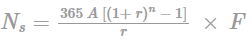A = Number of commercial vehicle or initial traffic = 1213 cvpd

r = Traffic growth rate = 8% per annum

n = design life = 2 years

f = vehicle damage factor = 2.5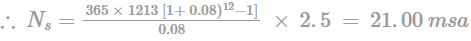Practice Test: Civil Engineering (CE)- 12 - Question 49

The velocity potential function in a two dimensional flow field is given by ∅ = x2-y2.The magnitude of velocity at point (1, 1) is

Detailed Solution for Practice Test: Civil Engineering (CE)- 12 - Question 49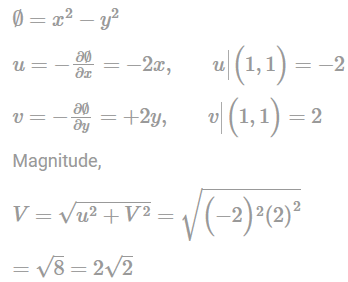Practice Test: Civil Engineering (CE)- 12 - Question 50

The flow in a rectangular channel is subcritical. If width of the channel is reduced at a certain section, the water surface under no–choke condition will

Detailed Solution for Practice Test: Civil Engineering (CE)- 12 - Question 50

In the subcritical region of flow for a given specific energy, as the discharge per unit width q is increased due to reducing width at the section, the depth of flow will decrease.

Therefore the water surface drops at a downstream section.

Alternately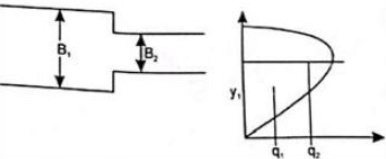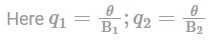∴ q1 > q2

From the discharge diagram, it can be seen that, for subcritical flow as q increases, depth decreases.

Therefore at the reduced section, depth decreases.

Practice Test: Civil Engineering (CE)- 12 - Question 51

Given, three spans each of length 2 m.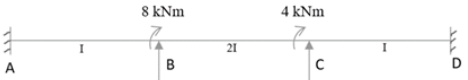What will be the change in rotation of θB if the middle span settles by 4/EI.

Detailed Solution for Practice Test: Civil Engineering (CE)- 12 - Question 51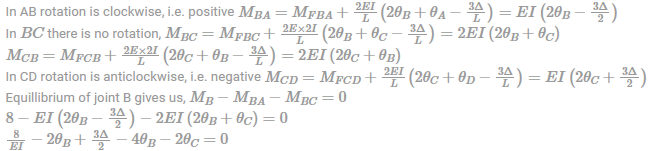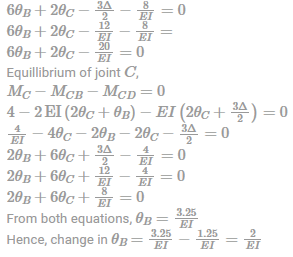Practice Test: Civil Engineering (CE)- 12 - Question 52

A thin gas cylinder with an internal radius of 100 mm is subject to an internal pressure of 10 MPa. The maximum permissible working stress is restricted to 100 MPa. The minimum cylinder wall thickness (in mm) for safe design must be

Detailed Solution for Practice Test: Civil Engineering (CE)- 12 - Question 52

We know that the σ1 = pd/2t

Putting values, 100 = 10×2×100/2×t

⇒ t = 100 mm

Practice Test: Civil Engineering (CE)- 12 - Question 53

In a preliminary survey a line of level was run from a bench mark of RL 454.650m and following readings are obtained. fly levelling was done till last position of the instrument

2.545,1.365,3.865,2.945,2.670,1.885,1.125

From the last position of the instrument five pegs at 20m interval and to be set out ib an uniformly rising gradient of 1 in 40. The first peg is to have a Rl of 455.110 Find the staff reading for the fourth peg.

Detailed Solution for Practice Test: Civil Engineering (CE)- 12 - Question 53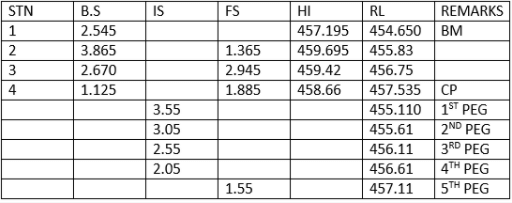*Answer can only contain numeric values
Practice Test: Civil Engineering (CE)- 12 - Question 54

What is the value of friction factor, if the diameter of pipe is 100 cm and roughness height is 0.25 cm?

Detailed Solution for Practice Test: Civil Engineering (CE)- 12 - Question 54 Friction factor for turbulent flow in rough pipe is given by

1/√f = 2 log (R/K) + 1.74

f = 0.0248

*Answer can only contain numeric values
Practice Test: Civil Engineering (CE)- 12 - Question 55

The specific gravity & weight proportion of aggregate & bitumen are as under for the preparation of Marshall Mix design. The volume & weight of one marshal specimen was found to be 600 cc and 1000 gm. Assuming absorption of bitumen in aggregate is zero. The percentage air void is ________%.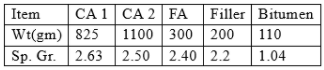Detailed Solution for Practice Test: Civil Engineering (CE)- 12 - Question 55 Phase Diagram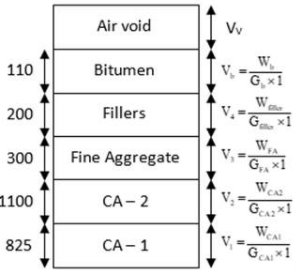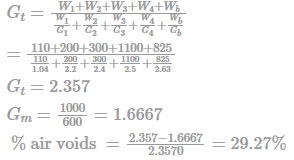Practice Test: Civil Engineering (CE)- 12 - Question 56

The driver of a vehicle travelling 75 km/hr up a gradient requires 10 m less to stop after he applied brakes, as compared to a driver travelling at the same speed, down the same gradient given, f = 0.40 what is the present gradient?

Detailed Solution for Practice Test: Civil Engineering (CE)- 12 - Question 56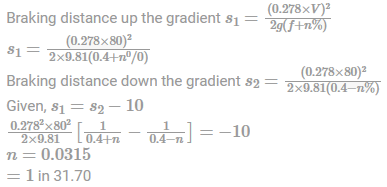Practice Test: Civil Engineering (CE)- 12 - Question 57

In a pipe of diameter 20 cm, a liquid of density 800 kg/m3 is flowing with a discharge of pipe 350 l/sec and friction factor is estimated as 0.0085. If viscosity of liquid is 2.9×10-4 Ns/m2 and assuming pipe is in smooth turbulent flow region then shear velocity will be

Detailed Solution for Practice Test: Civil Engineering (CE)- 12 - Question 57

Given ; d = 20 cm ; ρ = 800 kg/m3 ; Q = 350 l/sec = 0.35 m3/sec

f = 0.0085 ; µ = 2.9 × 10–4 Ns/m2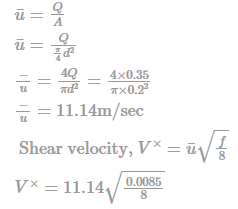V× = 0.363 m/sec

Practice Test: Civil Engineering (CE)- 12 - Question 58

What are the coordinates of the center of Mohr’s circle for an element subjected to two mutually perpendicular stresses one tensile of magnitude 80MPa and other compressive of magnitude 50MPa?

Detailed Solution for Practice Test: Civil Engineering (CE)- 12 - Question 58

σx = 80 MPa,

σy = -50 MPa

Co-ordinates of center of Mohr’s circle =[ ½( σx + σy),0]

= [(30/2),0]

= (15,0)

Practice Test: Civil Engineering (CE)- 12 - Question 59

Determine the deoxygenation coefficient at 37˚C, when the self-purification factor of a river is 4.25 and the rate at which re-oxygenation happens is 0.3 per day at a standard temperature of 20˚C.

Detailed Solution for Practice Test: Civil Engineering (CE)- 12 - Question 59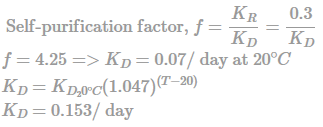Practice Test: Civil Engineering (CE)- 12 - Question 60

When two mutually perpendicular principal stresses are unequal but alike, the maximum shear is represented by __________.

Detailed Solution for Practice Test: Civil Engineering (CE)- 12 - Question 60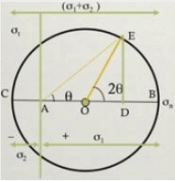It's half of the dia. Shows the maximum stress at angle is 45 so radius of the circle shows the maximum stress.

Practice Test: Civil Engineering (CE)- 12 - Question 61

To lift water from a ground reservoir to an overhead tank of capacity 0.90 cu.m. capacity, what is the appropriate H.P. of centrifugal pump to be installed, such that the OHT gets filled up in 1 hour. Consider the difference in water level as 50m. Assume the efficiency as 65%.

Detailed Solution for Practice Test: Civil Engineering (CE)- 12 - Question 61

Total head, H = 4m + 50m = 54m

Brake Horsepower of Pump (in H.P.) = wQH/75n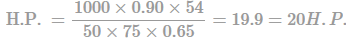*Answer can only contain numeric values
Practice Test: Civil Engineering (CE)- 12 - Question 62

In a typical load cycle over a component the maximum and minimum stresses of 245 N/m2 and 132 N/m2 are indicated respectively. Therefore the variable stress induced is _________.

Detailed Solution for Practice Test: Civil Engineering (CE)- 12 - Question 62 Given : σmax = 245 N/m2

σmin = 132 N/m2

The variable stress is given by,

Variable stress, σv = σmax − σmin/2 = 245−132/2 = 56.5MPa

*Answer can only contain numeric values
Practice Test: Civil Engineering (CE)- 12 - Question 63

The plastic limit of soil is 25%. Ir = 6%, where the soil is dried from its state at plastic limit, the volume change is 25% of its value at plastic limit. The corresponding change from liquid state to dry state is 30% of its volume at liquid limit. Determine the shrinkage ratio:

Detailed Solution for Practice Test: Civil Engineering (CE)- 12 - Question 63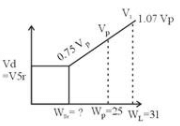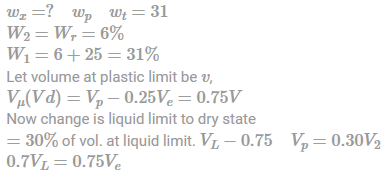Now equating the slopes: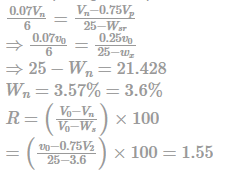Practice Test: Civil Engineering (CE)- 12 - Question 64

A cantilever beam of square cross section having depth 600 mm and span 8 m is subjected to a uniformly distributed load of magnitude 8.25 kN/m2. The stress generated at a distance equal to 2m from the free end and at a depth of 250 mm from top will be

Detailed Solution for Practice Test: Civil Engineering (CE)- 12 - Question 64

Bending moment at a distance equal to 2 m =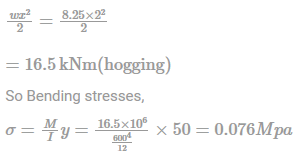Since the bending moment is hogging in nature, stresses above the neutral axis will be tensile in nature.

Practice Test: Civil Engineering (CE)- 12 - Question 65

The necessary and sufficient condition for a surface to be called as a free surface is

Detailed Solution for Practice Test: Civil Engineering (CE)- 12 - Question 65

In physics, a free surface is the surface of a fluid that is subject to zero parallel shear stress, such as the boundary between two homogeneous fluids, for example liquid water and the air in the Earth's atmosphere. Unlike liquids, gases cannot form a free surface on their own. So, no stress should be acting on it.

## GATE Civil Engineering (CE) 2023 Mock Test Series

27 docs|296 tests
 Use Code STAYHOME200 and get INR 200 additional OFF Use Coupon Code
Information about Practice Test: Civil Engineering (CE)- 12 Page
In this test you can find the Exam questions for Practice Test: Civil Engineering (CE)- 12 solved & explained in the simplest way possible. Besides giving Questions and answers for Practice Test: Civil Engineering (CE)- 12, EduRev gives you an ample number of Online tests for practice

## GATE Civil Engineering (CE) 2023 Mock Test Series

27 docs|296 tests